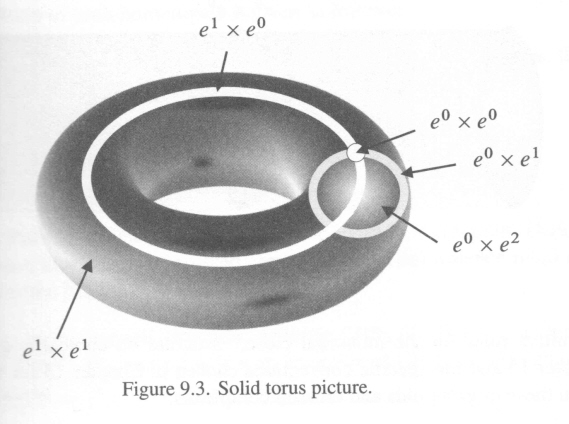# Product of CW complexes question

I am having trouble understanding the product of CW complexes. I know how to actually do the computations and all, I just don't understand how exactly it works.

So here's my questions specifically: If $X,Y$ are CW-complexes then say $e,f$ are $p,q$ cells on $X,Y$ respectively, then we know that $e \times f$ will be a $p+q$ cell in $X \times Y$. But this cell we have to think of as $D^{p+q}$ with some identification on the boundary sphere. But what we have here is $(D^p \times D^q)$. So I guess we need to know that we have homeomorphism of pairs $(D^{p+q},S^{p+q-1}) \cong (D^p \times D^q, S^{p-1} \times D^q \cup D^p \times S^{q-1})$. But that's what I do not get. How exactly do this homeomorphism work?

I kinda see it in the case $p=q=1$ (it's just that a square and a disc are homeomorphic with canonical identifications in the boundaries). But I am having trouble defining it or actually visualizing it in higher dimensions. Any help?

Thanks!

• I'm not sure I can help you visualize it in general, but we can at least manage with $p = 2$ and $q = 1$. Then $D^2 \times D^1$ is a solid cylinder, and the boundary is $S^1 \times D^1$, a vertical tube, together with $D^2 \times S^0$, the two end caps. The boundary operator on manifolds with boundary is a derivation in this fashion in general: $\partial(M \times N) = (\partial M \times N) \cup (M \times \partial N)$. – jdc May 13 '14 at 23:08
• Oh OK. So you're saying that just because $D^{p+q} \cong D^p \times D^q$ the iso on the boundaries will follow? Huh that was really simple after all! Thanks! – Bernard May 13 '14 at 23:26
• If you think of it in terms of manifolds with boundary/corners, the points in the product that don't have open ball neighborhoods have to have at least one coordinate on the boundary of one of the factor manifolds. – jdc May 13 '14 at 23:29

## 2 Answers

You can think of $D^n$ as the homeomorphic cube $I^n$. This way, the product $$\left(D^k\times D^l,\ \partial D^k× D^l\cup D^k×∂D^l\right)\\ \cong\left(I^k×I^l,\ ∂I^k×I^l\cup I^k×∂I^l\right)\\ =\left(I^{k+l},∂\left(I^k×I^l\right)\right)\\ \cong \left(D^{k+l},∂\left(D^k×D^l\right)\right)$$

The homeomorphism between $D^k\cong I^k$ i given by $$x\mapsto \dfrac{x\cdot||x||_2}{||x||_\infty}$$

The characteristic map is $\Phi_{\alpha,\beta}=Φ_{α}×Φ_β:D^k×D^l\to X×Y$

• Note that since $X,Y$ are Hausdorff, so is $X\times Y$.
• The images of the interiors $Φ_{α,β}\left(\text{int}D^{k+l}\cong\text{int}D^k×\text{int}D^l\right)$ partition $X\times Y$.
• For each product cell $e_α×e_β$, the image of $∂\left(D^k×D^l\right)= ∂I^k×I^l\cup I^k×∂I^l$ is in finitely many cells of dimension less than $k+l$
• If the product topology on $X×Y$ is such that each set $A$ is closed if $A\cap \overline{e_{α,β}}$ is closed in $\overline{e_{α,β}}$ for each cell, then all conditions in the implicit definition are satisfied, so $X×Y$ will be a CW complex.
• The last condition generally not holds, but it holds for certain case like when either $X$ or $Y$ is locally compact. But I got stuck when proving it. Any hints? – Chen M Ling May 26 '16 at 18:52
• @ChenMLing The idea is that when $X\times Y$ is a $k$-space (also called compactly generated space), then the product topology is the topology as a CW complex, see math.stackexchange.com/questions/248639/…. This holds when $X$ or $Y$ is locally compact since the product of a $k$-space with a locally compact space is again a $k$-space. – Stefan Hamcke May 27 '16 at 0:35
• Yes. There is such theorem in Hatcher that the product of a locally compact space and a k-space is again a k-space. Since $X$ is a CW complex, then it is directly a k-space. But now the result gives $X\times Y$ is a k-space, this is where I got stuck: how to show that it is CW? – Chen M Ling May 27 '16 at 10:43
• See my answer for math.stackexchange.com/questions/248639/…. It shows that when $X\times Y$ is a $k$-space, then it is a CW complex. @ChenMLing – Stefan Hamcke May 27 '16 at 17:35
• Oh I missed that. Thanks @StefanHamcke – Chen M Ling May 28 '16 at 6:09

I hope the following picture of a solid torus with the cells labelled will help:It is taken from Nonabelian Algebraic Topology (pdf available there), which also compares algebraic modelling of products of cells as in your question and in terms of cubes; these are much easier to understand since the $m$-cube $I^m$ is the topological product of $m$ copies of the unit interval $I=[0,1]$ and so $I^{m+n} \cong I^m \times I^n$. The relation between cells and cubes is used a lot in algebraic topology. The natural cell structure of $I^m$ is more complicated than that of the $m$-cell $D^m= e^0 \cup e^{m-1} \cup e^m$, but you gain in other ways, so one needs both models.